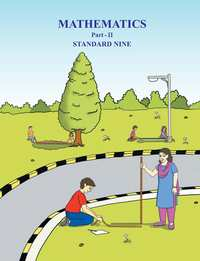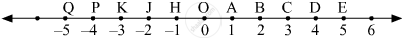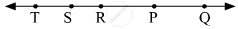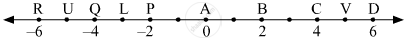Share

# Balbharati solutions for Maharashtra state board SSC 9th Standard Maths 2 Geometry chapter 1 - Basic Concepts in Geometry [Latest edition]

Textbook page

#### Chapters## Chapter 1: Basic Concepts in Geometry

Practice set 1.1OthersPractice set 1.2Practice set 1.3Problem set 1

### Balbharati solutions for Maharashtra state board SSC 9th Standard Maths 2 Geometry Chapter 1 Basic Concepts in Geometry Practice set 1.1 [Page 5]

Practice set 1.1 | Q 1 | Page 5

Find the distances with the help of the number line given below.(i) d(B,E)
(ii) d(J, A)
(iii) d(P, C)
(iv) d(J, H)
(v) d(K, O)
(vi) d(O, E)
(vii) d(P, J)
(viii) d(Q, B)

Practice set 1.1 | Q 2.1 | Page 5

If the co-ordinate of A is x and that of B is y, find d(A, B).

x = 1, y = 7

Practice set 1.1 | Q 2.2 | Page 5

If the co-ordinate of A is x and that of B is y, find d(A, B).

x = 6, y = - 2

Practice set 1.1 | Q 2.3 | Page 5

If the co-ordinate of A is x and that of B is y, find d(A, B).

x = - 3, y = 7

Practice set 1.1 | Q 2.4 | Page 5

If the co-ordinate of A is x and that of B is y, find d(A, B).

x = - 4, y = - 5

Practice set 1.1 | Q 2.5 | Page 5

If the co-ordinate of A is x and that of B is y, find d(A, B).

x = - 3, y = - 6

Practice set 1.1 | Q 2.6 | Page 5

If the co-ordinate of A is x and that of B is y, find d(A, B).

x = 4, y = - 8

Practice set 1.1 | Q 3.1 | Page 5

From the information given below, find which of the point is between the other two. If the points are not collinear, state so.

d(P, R) = 7,   d(P, Q) = 10,   d(Q, R) = 3

Practice set 1.1 | Q 3.2 | Page 5

From the information given below, find which of the point is between the other two.
If the points are not collinear, state so.

d(R, S) = 8, d(S, T) = 6, d(R, T) = 4

Practice set 1.1 | Q 3.3 | Page 5

From the information given below, find which of the point is between the other two.
If the points are not collinear, state so.

d(A, B) = 16,  d(C, A) = 9,   d(B, C) = 7

Practice set 1.1 | Q 3.4 | Page 5

From the information given below, find which of the point is between the other two.
If the points are not collinear, state so.

d(L, M) = 11,   d(M, N) = 12,   d(N, L) = 8

Practice set 1.1 | Q 3.5 | Page 5

From the information given below, find which of the point is between the other two.
If the points are not collinear, state so.

d(X, Y) = 15,   d(Y, Z) = 7,   d(X, Z) = 8

Practice set 1.1 | Q 3.6 | Page 5

From the information given below, find which of the point is between the other two.
If the points are not collinear, state so.

d(D, E) = 5,   d(E, F) = 8,   d(D, F) = 6

Practice set 1.1 | Q 4 | Page 5

On a number line, points A, B and C are such that d(A,C) = 10, d(C,B) = 8 .  Find d(A, B) considering all possibilities.

Q 5 | Page 5

Points X, Y, Z are collinear such that d(X,Y) = 17, d(Y,Z) = 8, find d(X,Z) .

Practice set 1.1 | Q 6.1 | Page 5
Sketch proper figure and write the answer of the following question.
If A - B - C   and   l(AC) = 11,  l(BC) = 6.5,   then l(AB) =?
Practice set 1.1 | Q 6.2 | Page 5
Sketch proper figure and write the answer of the following question.
If R - S - T   and   l(ST) = 3.7,   l(RS) = 2.5,   then l(RT) =?
Practice set 1.1 | Q 6.3 | Page 5
Sketch proper figure and write the answer of the following question.
If X - Y - Z  and   l(XZ) = 3√7, l(XY) = √7,  then l(YZ) =?
Practice set 1.1 | Q 7 | Page 5

Which figure is formed by three non-collinear points?

### Balbharati solutions for Maharashtra state board SSC 9th Standard Maths 2 Geometry Chapter 1 Basic Concepts in Geometry Practice set 1.2 [Pages 7 - 8]

Practice set 1.2 | Q 1 | Page 7

The following table shows points on a number line and their co-ordinates. Decide whether the pair of segments given below the table are congruent or not.

 Point A B C D E Co-ordinate 3 5 2 7 9

(i) seg DE and seg AB

(ii) seg BC and seg AD

(iii) seg BE and seg AD

Practice set 1.2 | Q 2 | Page 7

Point M is the midpoint of seg AB. If AB = 8 then find the length of AM.

Practice set 1.2 | Q 3 | Page 7

Point P is the midpoint of seg CD. If CP = 2.5, find l(CD).

Practice set 1.2 | Q 4 | Page 7

If AB = 5 cm, BP = 2 cm and AP = 3.4 cm, compare the segments.

Practice set 1.2 | Q 5 | Page 8

Write the answers to the following questions with reference in the given figure.(i) Write the name of the opposite ray of ray RP.
(ii) Write the intersection set of ray PQ and ray RP.
(iii) Write the union set of ray PQ and ray QR.
(iv) State the rays of which seg QR is a subset.
(v) Write the pair of opposite rays with common end point R.
(vi) Write any two rays with common end point S.
(vii) Write the intersection set of ray SP and ray ST.
Practice set 1.2 | Q 6 | Page 8

Answer the questions with the help of  a given figure.(i) State the points which are equidistant from point B.
(ii) Write a pair of points equidistant from point Q.
(iii) Find d(U,V), d(P,C), d(V,B),d(U, L).

### Balbharati solutions for Maharashtra state board SSC 9th Standard Maths 2 Geometry Chapter 1 Basic Concepts in Geometry Practice set 1.3 [Page 11]

Practice set 1.3 | Q 1.1 | Page 11

Write the following statement in ‘if-then’ form.

The opposite angles of a parallelogram are congruent.

Practice set 1.3 | Q 1.2 | Page 11

Write the following statement in ‘if-then’ form.

The diagonals of a rectangle are congruent.

Practice set 1.3 | Q 1.3 | Page 11
Write the following statement in ‘if-then’ form.
In an isosceles triangle, the segment joining the vertex and the mid point of the base is perpendicular to the base.

Practice set 1.3 | Q 2.1 | Page 11

Write converses of the following statement.

The alternate angles formed by two parallel lines and their transversal are congruent.

Practice set 1.3 | Q 2.2 | Page 11

Write converses of the following statement.

If a pair of the interior angles made by a transversal of two lines are supplementary then the lines are parallel.

Practice set 1.3 | Q 2.3 | Page 11

Write converses of the following statement.

The diagonals of a rectangle are congruent.

### Balbharati solutions for Maharashtra state board SSC 9th Standard Maths 2 Geometry Chapter 1 Basic Concepts in Geometry Problem set 1 [Pages 11 - 12]

Problem set 1 | Q 1.1 | Page 11

Select the correct alternative from the answer of the question given below.

How many mid points does a segment have?

•  only one

• two

• three

• many

Problem set 1 | Q 1.2 | Page 11
Select the correct alternative from the answer of the question given below.
How many points are there in the intersection of two distinct lines?
• infinite

• two

• one

• not a single

Problem set 1 | Q 1.3 | Page 11
Select the correct alternative from the answer of the question given below.
How many lines are determined by three distinct points?
• two

• three

• one or three

• six

Problem set 1 | Q 1.4 | Page 11
Select the correct alternative from the answer of the question given below.
Find d(A, B), if co-ordinates of A and B are -2 and 5 respectively.
• -2

• 5

• 7

• 3

Problem set 1 | Q 1.5 | Page 11
Select the correct alternative from the answer of the question given below.
If P - Q - R and d(P,Q) = 2, d(P,R) = 10, then find d(Q,R)
• 12

• 8

• sqrt(96)

• 20

Problem set 1 | Q 2.1 | Page 11

On a number line, co-ordinates of P, Q, R are 3, -5 and 6 respectively. State with reason whether the following statement is true or false.

d(P,Q) + d(Q,R) = d(P,R)

• True

• False

Problem set 1 | Q 2.2 | Page 11

On a number line, co-ordinates of P, Q, R are 3, -5 and 6 respectively. State with reason whether the following statement is true or false.

d(P,R) + d(R,Q) = d(P,Q)

• True

• False

Problem set 1 | Q 2.3 | Page 11

On a number line, co-ordinates of P, Q, R are 3, -5 and 6 respectively. State with reason whether the following statements are true or false.

d(R,P) + d(P,Q) = d(R,Q)

• True

• False

Problem set 1 | Q 2.4 | Page 11

On a number line, co-ordinates of P, Q, R are 3, -5 and 6 respectively. State with reason whether the following statements are true or false.

d(P,Q) - d(P,R) = d(Q,R)

• True

• False

Problem set 1 | Q 3.1 | Page 11

Co-ordinates of the pair of a point is given below. Hence find the distance between the pair.

3, 6

Problem set 1 | Q 3.2 | Page 11

Co-ordinates of the pair of points are given below. Hence find the distance between the pair.

-9, -1

Problem set 1 | Q 3.3 | Page 11

Co-ordinates of the pair of points are given below. Hence find the distance between the pair.

-4, 5

Problem set 1 | Q 3.4 | Page 11

Co-ordinates of the pair of points are given below. Hence find the distance between the pair.

x, -2

Problem set 1 | Q 3.5 | Page 11

Co-ordinates of the pair of points are given below. Hence find the distance between the pair.

x + 3, x - 3

Problem set 1 | Q 3.6 | Page 11

Co-ordinates of the pair of points are given below. Hence find the distance between the pair.

-25, -47

Problem set 1 | Q 3.7 | Page 11

Co-ordinates of the pair of points are given below. Hence find the distance between the pair.

80, - 85

Problem set 1 | Q 4 | Page 12

Co-ordinate of point P on a number line is - 7. Find the co-ordinates of points on the number line which are at a distance of 8 units from point P.

Problem set 1 | Q 5.1 | Page 12

If A - B - C and d(A,C) = 17, d(B,C) = 6.5 then d(A,B) = ?

Problem set 1 | Q 5.2 | Page 12

If P - Q - R and d(P,Q) = 3.4, d(Q,R)= 5.7 then d(P,R) = ?

Problem set 1 | Q 6 | Page 12

Co-ordinate of point A on a number line is 1. What are the co-ordinates of points on the number line which are at a distance of 7 units from A?

Problem set 1 | Q 7.1 | Page 12
Write the following statement in conditional form.
Every rhombus is a square.
Problem set 1 | Q 7.2 | Page 12

Write the following statement in conditional form.

Angles in a linear pair are supplementary.

Problem set 1 | Q 7.3 | Page 12

Write the following statement in conditional form.

A triangle is a figure formed by three segments.

Problem set 1 | Q 7.4 | Page 12

Write the following statement in conditional form.

A number having only two divisors is called a prime number.

Problem set 1 | Q 8.1 | Page 12
Write the converse of the following statement.
If the sum of measures of angles in a figure is 1800 , then the figure is a triangle.
Problem set 1 | Q 8.2 | Page 12

Write the converse of the following statement.

If the sum of measures of two angles is 900 then they are complement of each other.

Problem set 1 | Q 8.3 | Page 12

Write the converse of the following statement.

If the corresponding angles formed by a transversal of two lines are congruent then the two lines are parallel.

Problem set 1 | Q 8.4 | Page 12

Write the converse of the following statement.

If the sum of the digits of a number is divisible by 3 then the number is divisible by 3.

Problem set 1 | Q 9.1 | Page 12

Write the antecedent (given part) and the consequent (part to be proved) in the following statement.

If all sides of a triangle are congruent then its all angles are congruent.

Problem set 1 | Q 9.2 | Page 12

Write the antecedent (given part) and the consequent (part to be proved) in the following statement.

The diagonals of a parallelogram bisect each other.

Problem set 1 | Q 10.1 | Page 12
Draw a labelled figure showing information in the following statement and write the antecedent and the consequent.
Two equilateral triangles are similar.

Problem set 1 | Q 10.2 | Page 12
Draw a labelled figure showing information in the following statement and write the antecedent and the consequent.
If angles in a linear pair are congruent then each of them is a right angle.
Problem set 1 | Q 10.3 | Page 12
Draw a labelled figure showing information in the following statement and write the antecedent and the consequent.
If the altitudes drawn on two sides of a triangle are congruent then those two sides are congruent.

## Chapter 1: Basic Concepts in Geometry

Practice set 1.1OthersPractice set 1.2Practice set 1.3Problem set 1## Balbharati solutions for Maharashtra state board SSC 9th Standard Maths 2 Geometry chapter 1 - Basic Concepts in Geometry

Balbharati solutions for Maharashtra state board SSC 9th Standard Maths 2 Geometry chapter 1 (Basic Concepts in Geometry) include all questions with solution and detail explanation. This will clear students doubts about any question and improve application skills while preparing for board exams. The detailed, step-by-step solutions will help you understand the concepts better and clear your confusions, if any. Shaalaa.com has the Maharashtra State Board Maharashtra state board SSC 9th Standard Maths 2 Geometry solutions in a manner that help students grasp basic concepts better and faster.

Further, we at Shaalaa.com provide such solutions so that students can prepare for written exams. Balbharati textbook solutions can be a core help for self-study and acts as a perfect self-help guidance for students.

Concepts covered in Maharashtra state board SSC 9th Standard Maths 2 Geometry chapter 1 Basic Concepts in Geometry are Basic Concept in Geometry, Co-ordinates of points and distance, Basic concept of segment, ray and line, Conditional Statements and Converse, Proofs.

Using Balbharati Class 9 solutions Basic Concepts in Geometry exercise by students are an easy way to prepare for the exams, as they involve solutions arranged chapter-wise also page wise. The questions involved in Balbharati Solutions are important questions that can be asked in the final exam. Maximum students of Maharashtra State Board Class 9 prefer Balbharati Textbook Solutions to score more in exam.

Get the free view of chapter 1 Basic Concepts in Geometry Class 9 extra questions for Maharashtra state board SSC 9th Standard Maths 2 Geometry and can use Shaalaa.com to keep it handy for your exam preparation